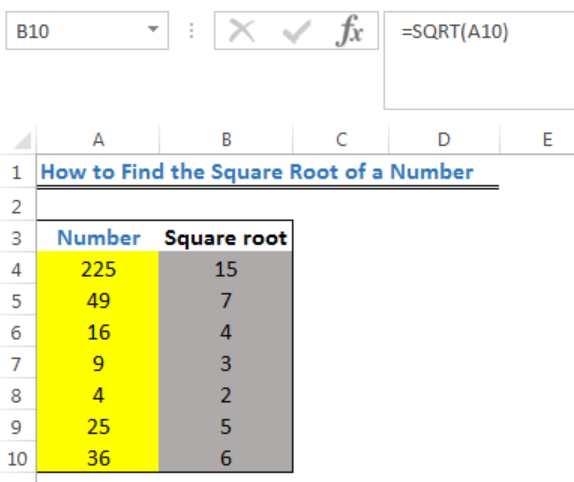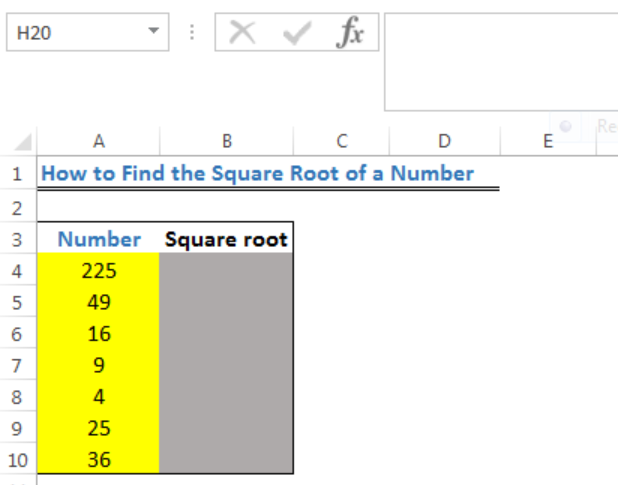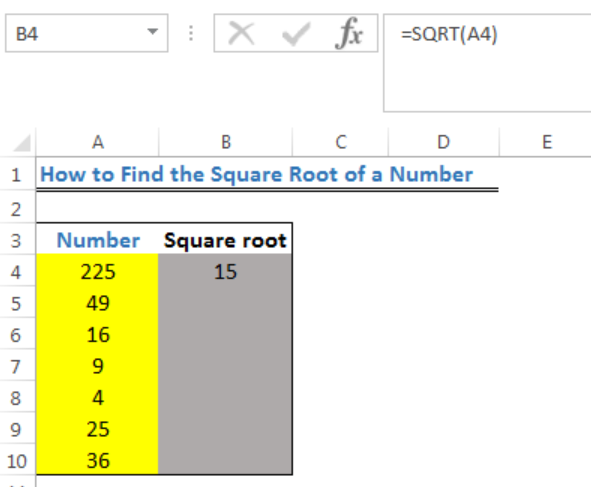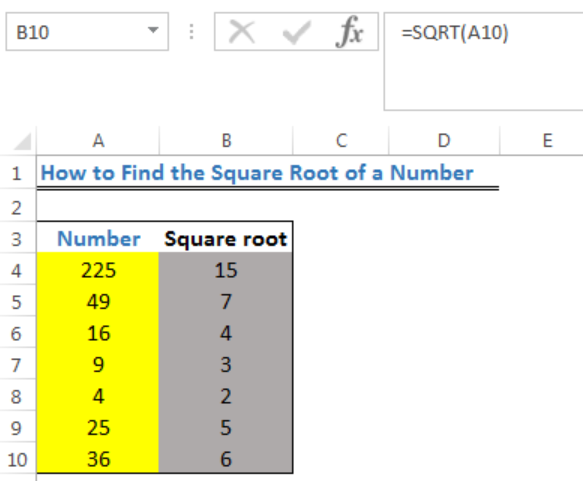Get instant live expert help with Excel or Google Sheets“My Excelchat expert helped me in less than 20 minutes, saving me what would have been 5 hours of work!”

#### Post your problem and you’ll get expert help in seconds.

Your message must be at least 40 characters
Our professional experts are available now. Your privacy is guaranteed.

# How to Find the Square Root of a Number

We can find the SQUARE ROOT of a number with either the SQRT function or the POWER function with the corresponding power argument (1/2). The steps below will walk through the process.Figure 1: How to Find the Square Root of a Number

## Formula

SQRT function: `=SQRT(cell containing the number or the number)`

POWER function: `=POWER(cell containing the number or the number,1/2)`

## Setting up the Data

• We will set up the data as shown in figure 2
• The numbers whose square root we intend to find are in Cell A4 to Cell A10
• The results will be returned in Column BFigure 2: Setting up the Data

## Find the Square Root of a Number

• We will click on Cell B4
• We will insert the formula below:
`=SQRT(A4)`
• We will press the enter key to get the resultFigure 3: Square Root for the Value in Cell A4

• We will use the fill handle to copy the formula into the other cellsFigure 4: Square Root of the Numbers in Column A

## Note

To use the POWER FUNCTION, enter the formula below into Cell B4 and repeat the process above.

Formula: `=POWER(A4,1/2)`

## Instant Connection to an Expert through our Excelchat Service

Most of the time, the problem you will need to solve will be more complex than a simple application of a formula or function. If you want to save hours of research and frustration, try our live Excelchat service! Our Excel Experts are available 24/7 to answer any Excel question you may have. We guarantee a connection within 30 seconds and a customized solution within 20 minutes.

### Did this post not answer your question? Get a solution from connecting with the expert.Another blog reader asked this question today on Excelchat:
Related blogs
Solution examplesI need help with solving equations using separate columns. For example. One column has pH values going from 0-14 and the column next to it has the equation [H+] = 10^-pH with it's corresponding values.
Solved by I. J. in 18 mins## Subscribe to Excelchat.coAnother blog reader asked this question today on Excelchat: